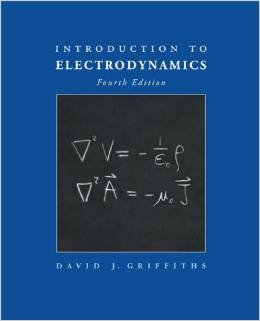PHYSICS 218: Electromagnetism II

Electromagnetic induction; displacement current; Maxwell's equations; the wave equation; plane electromagnetic waves; Poynting vector; reflection and refraction; radiation; waveguides; transmission lines; propagation of light; radiation by charged particles; relativistic formulation of Maxwell's equations.

PREREQUISITES: PHY217, PHY 123 or PHY 143; MTH 281 (may be taken concurrently).

The class will use: Introduction of Electrodynamics (4th Ed.) by David J. Griffiths